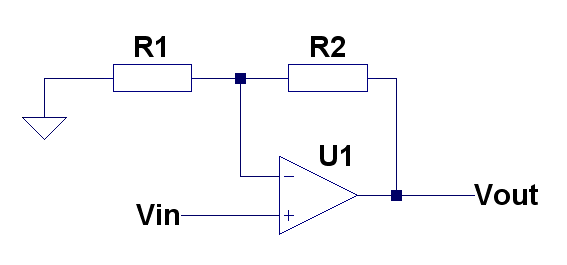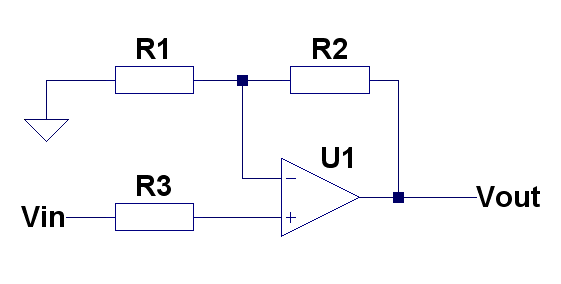# Non-inverting amplifierVout =Vin* (R1+R2)/R1
or
Vout =Vin* (1+R2/R1)

Gain = (R1+R2)/R1
or
Gain = 1+R2/R1

## Canceling effect of input bias currentIf you want to be precise, R3 must be equal to R1||R2 . Or R3=(R1*R2)/(R1+R2).

If gain is quite high (10+) then you can use R3 = R1 because R2 will be too high to make significant difference.